# How to Use ISBLANK Function in Google Sheets

Learn how to use ISBLANK function in Google Sheets without getting FALSE results on blank cells. Important for data validation and analysis in your spreadsheet.Certain formulas in Google Sheets require the referenced cell to contain a value, else an error will show up...

We can prevent this from happening by adding a way to scan whether the referenced cell contains a value or not. One way is to use the ISBLANK function.

In this tutorial, we will learn how to use the ISBLANK function on one cell, on an array of cells (in two ways), and together with a formula that requires a value on the reference cell.

## ISBLANK on one cell

A handy function you can use to check whether a cell is empty or not (without clicking on it) is the ISBLANK function.  The ISBLANK function checks whether a cell is empty or not, returning either TRUE or FALSE:

=isblank(cell_reference)

It is useful because it actually allows you to check whether the cell is really empty or it contains either stray spaces or an apostrophe. The image below shows you how the result will look, depending on the contents of the cell.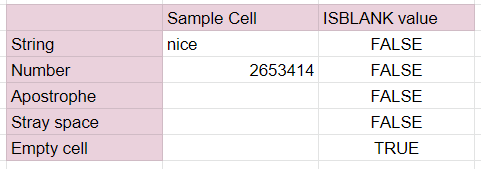A table showing the values ISBLANK returns for each type of data inside a cell. The ISBLANK function returns a FALSE value for cells containing a string, number, apostrophe, or stray space.

## ISBLANK on an array of cells

While ISBLANK also allows an array of cells as the reference, it has known issues when setting an array of cells as the reference of the formula, giving FALSE value even if the cell has no apostrophe nor stray spaces.

Besides, if we apply the ISBLANK function to an array of cells, we would want to see individually which cells are really empty or just appear empty. This is what we will learn in this section.

There are two ways to do so. One is to insert ISBLANK function on one cell, reference the first cell of the array, and then copy-drag that cell to cover the entire range: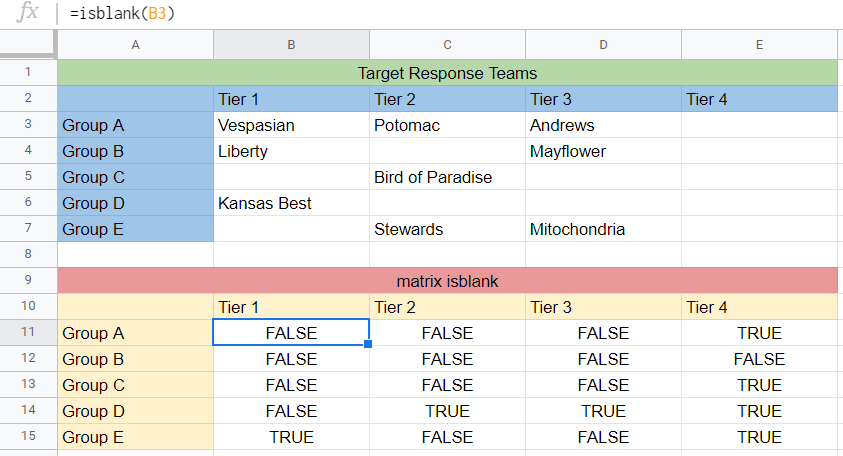Some cells that appear blank in the top array register a FALSE result in the bottom, indicating that they either have an apostrophe or stray spaces inside it.

ISBLANK can be combined with other functions. The other way to generate the same array as above is to put it as an argument inside ARRAYFORMULA and specify the range of the array as the reference of ISBLANK function:

=ARRAYFORMULA(isblank(B3:E7))

The result would look like the same: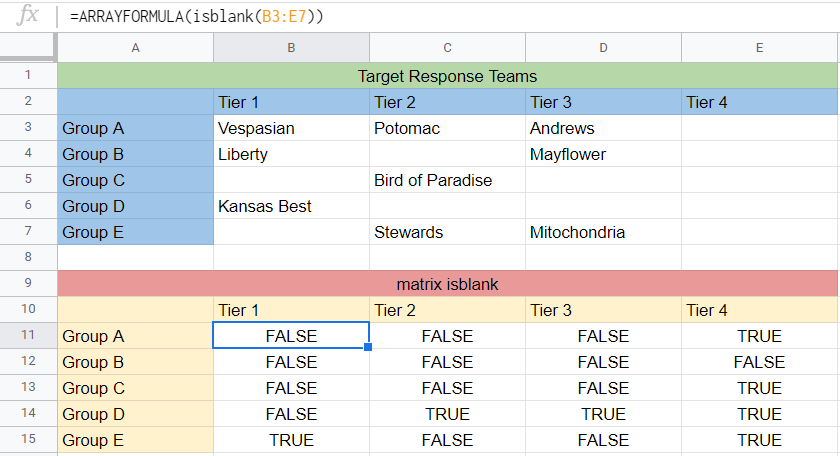The bottom array is now generated from using the ARRAYFORMULA function.

## ISBLANK on an array of cells, spiced up

We can further spice up our spreadsheet by combining ISBLANK function with IF function. With our same example above, we want the Google Sheets to print “Something’s written” if the cell contains something while it will print “Really empty” if the cell is indeed empty.

To implement that, we write the following formula:

=IF(ISBLANK(B3)=TRUE, "Really empty", "Something's written")

And our array will look like this: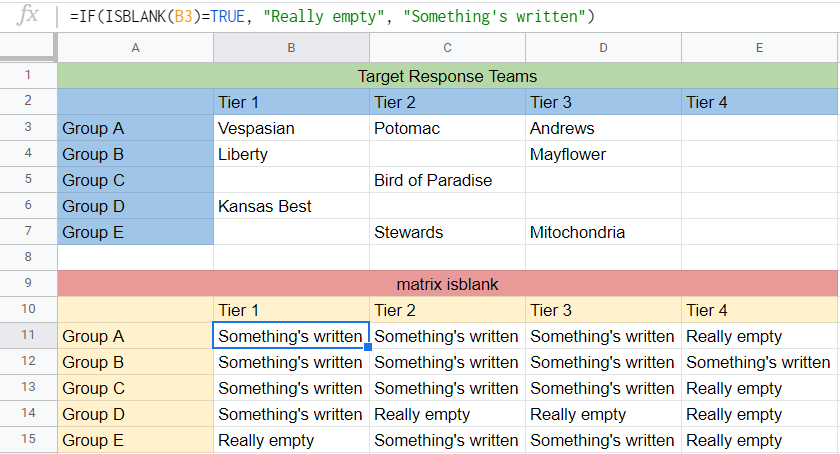The bottom array generated by encoding an IF function to display “Really empty” when the result of ISBLANK is TRUE, or display “Something’s written” when the result of ISBLANK is FALSE.

## ISBLANK with a formula that requires a cell with value

Finally we can now try using the ISBLANK to keep from getting an error from a formula that requires its reference cell to have a value.

For our final example, we wish to calculate the average sales per order of the teams listed. If either of the two values required, the total sales or the total number of orders is missing, the formula will not be able to correctly calculate the average sales per order metric.

We wish to have the formula indicate what is the missing quantity for each entry. To do so, we set the following conditions:

1. If the total sales is missing, the formula will display “Missing Total Sales”.
2. If the total number of orders is missing, the formula will display “Missing Total Orders”.
3. If both are present, the formula will calculate the average sales per order by dividing the total sales by the total orders.
4. If both are missing, the formula  will display “Missing Total Sales and Total Orders”.

To implement this, we will use the IFS function that allows multiple conditions. We set one condition and one result for each of the three given scenarios above. Our formula will look like this:

=IFS(AND(ISBLANK(H19)=TRUE,ISBLANK(I19)=FALSE),"Missing Total Sales",

AND(ISBLANK(H19)=FALSE,ISBLANK(I19)=TRUE),"Missing Total Orders",

AND(ISBLANK(H19)=FALSE,ISBLANK(I19)=FALSE),H19/I19,

AND(ISBLANK(H19)=TRUE,ISBLANK(I19)=TRUE),

"Missing Total Sales and Total Orders")

And the result will look like this: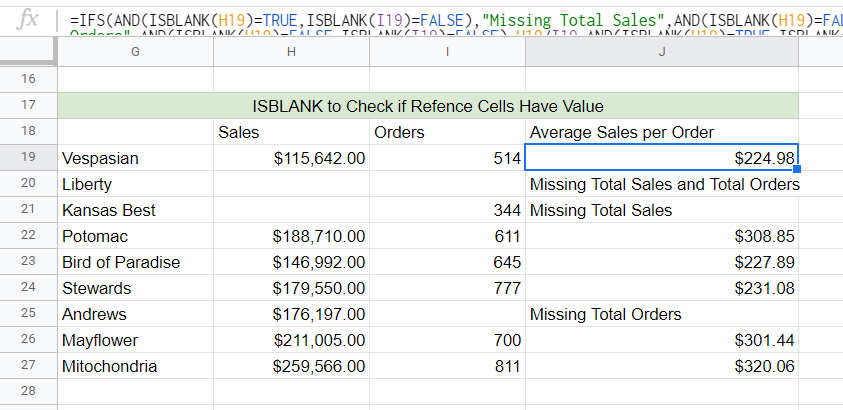The formula that combines the functionality of ISBLANK function with the versatility of IFS function. The function can scan the reference cells for possible lack of relevant data and display what is missing.

This is a pretty powerful combination of ISBLANK and IFS functions, but as you can see the formula already got quite long, with four conditions for two given reference cells. Nonetheless we have demonstrated how useful ISBLANK function is especially when combined with other functions in Google Sheets.

## A sample to further understand ISBLANK

To help you further understand how ISBLANK functions work, you can check the sample sheet here: ISBLANK Sample Sheet